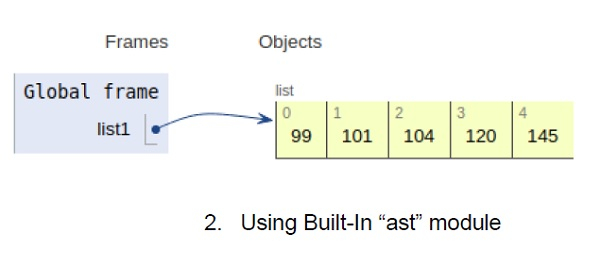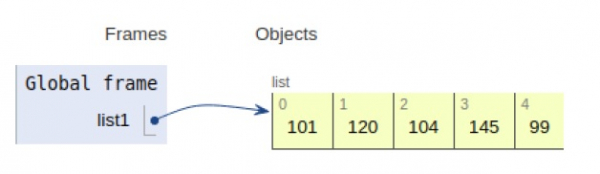# Python program to convert hex string to decimal

Problem statement − We are given a hexadecimal string, we need to convert it into its decimal equivalent.

We have two approaches to solve the problem−

• Brute-force Approach
• Using Built-in module

## Brute-Force Method

Here we take the help of explicit type casting function i.e. integer. This function takes two arguments i.e. hex equivalent and base i.e. (16). This function is used to convert a hexadecimal string to its equivalent decimal in an integer type, which can be further typecasted back to string format.

### Example

Live Demo

#input string
string = 'F'
# converting hexadecimal string to decimal
res = int(string, 16)
# print result
print("The decimal number associated with hexadecimal string is : " + str(res))

### Output

The decimal number associated with hexadecimal string is: 15## Using Built-In “ast” module

Here we use literal_eval function available in ast module, we can predict the base of given hexadecimal equivalent and then convert into its decimal equivalent. Here we use the concept of literal evaluation.

### Example

Live Demo

# using built-in module literal_eval
from ast import literal_eval
# initializing string
test_string = '0xF'
# converting hexadecimal string to decimal
res = literal_eval(test_string)
# print result
print("The decimal number of the hexadecimal string is : " + str(res))

### Output

The decimal number of the hexadecimal string is: 15All the variables are declared in the local scope and their references are seen in the figure above.